# Difference between while and do while loop

In any programming language, whenever we are interested in implementing a single statement multiple times i.e. if we want to type a number for specific number of times or until a specified condition is fulfilled, we do not write the statement that number of times rather we use loops like whiledo-while and for loops. As the name suggest a loop is like a goto statement, it will execute a particular block of statements and then go up to the start of the loop definition. They will continue to do so for a specific number of times (for loops) and they will also loop back to the start until a specific Boolean condition is fulfilled (while loops or do while loops). Let’s broadly divide the loops in programming languages in some types.

## Types of loops

• For next loops
• While loops (conditional loop)
• Do while loops (conditional loop)

Now I will explain the use and purpose of each of them and after that we will move towards major difference between while and do while loops.

## For next loops

For next loop are the simplest loops to work with in iterative loops or implementation of iterative statement in their logical explanation. However, the implementation of for loops a bit more complex than while loops the complexity level of implementation and understanding go in the flow like given below,

While loops < Do while loop < For next loop

Less complex – – – – – – – – – – – – – – – – – – – – – – – – – – – – – – > more complex

The starting statement of a for next loop contains a variable initialization, a conditional statement and an incremental statement. The first (initialization) statement initialize a variable to some value, this variable is going to be used inside the for-loop block. The second statement contains a conditional statement which contains the same initialized variable and the last statement increments the variable to some base case so that the conditional statement converges. After the main statement comes the body of the for next loop, which contains the statement or multiple statements which we want to be executed iteratively. The body of a for next loop is provided below,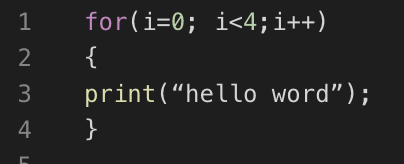Figure 1: for loop body

This for loop will print help world 4 times in the same line.

The flowchart of the for next loop is shown in the figure below,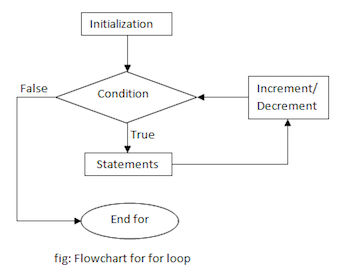Figure 2: Flowchart of for loop

Lets’ divert our concentration towards our main topic which is the implementation of conditional loops i.e. while loop and do while loop

## While loops

Now come the simplest loops to implement in any programming languages, the implementation or body of a while loop contains only 2 parts. One part is the main statement or conditional line which contains the Boolean conditional statement in which needs to be satisfied in order to implement the statements inside the while block, and the second part contains the while blocks statements itself, i.e. one or more statements which we want to implement as long as the given condition in first part is satisfied. The body or implementation of a conditional while loop is shown below,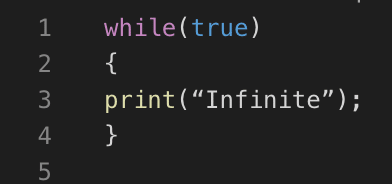Figure 3: while loop block

This is an infinite while loop and it will print “infinite” infinite times. These kind of loops are using where we have a hardware to stop the processing externally, whereas in loops which has to converge to some base case these conditions need to be valid which and there must be a scenario under which the conditional statement no longer satisfies so that the control may come out of the loop to complete the program execution. And the convergence of base case must lie inside the while loop. A perfect code for while loop implementation is shown in the figure below,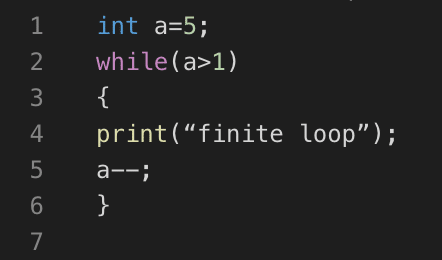Figure 4: finite while loop

This code will print “finite loop” 4 times as after that the condition of while loop no longer supports logic.

The flow chart of implementation of a while loop is shown in the figure below,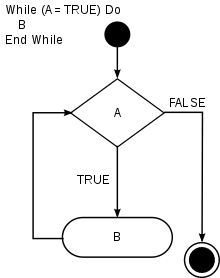Figure 5: while loop

We can also compare it with if else statement i.e. if the condition in the while statement is true it will continue execution the processing of same while block else it will stop the execution. The simple example of while loop implemented in java language is shown in the image below,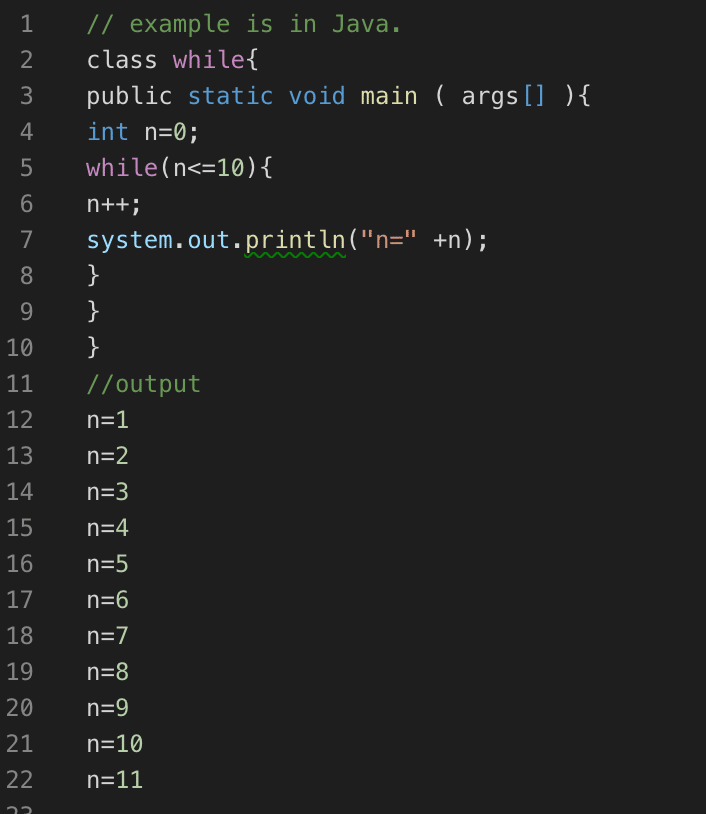Figure 6: While loop implementation

In the start the variable n is initialized as n=0 and the while loop is checked for a condition n<=10 which is true. Inside of while loop there is a statement which converges the value of n towards the condition i.e. n=10, which is n++ this statement increments the value of n at every iteration of loop and in the same block there is a statement which prints the value of n after its increment. Hence the output of the while loop is shown in the above figure.

## Do while loop

Do while loops are not very different from while loops except for one thing. Before jumping to the difference let’s first discuss the block elements of a do while look. A do while loop block consists of 3 parts. The first part is a do keyword which is used before the main code block. After that comes the code block, in the code block we write statements which are to be executed, and the third part is the while and conditional part which contains a while keyword and a condition which needs to be satisfied in order to reenter the code block. The figure below shows the body of a do while loop.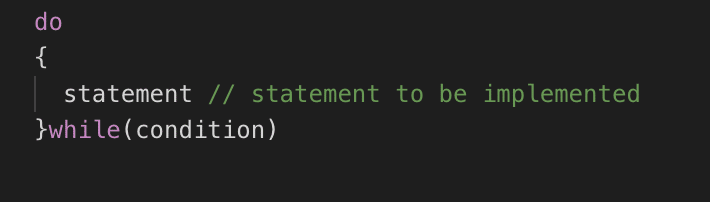Figure 7: Do while loop body

One major difference between while loop and do while is that in while loop the code block only be implemented if the while condition is satisfied and if it’s not the code will simply skip the while loop and move to the statement next to while loop. In simple word the condition will be tested before the implementation of code block.

In do while loop the code section will be executed once, and after one iteration, the control will check the while condition if it’s true than the second iteration will be executed, else the control will execute the statement next to do while loop.

Summarizing this whole discussion, while loop will not execute the code block if statement is false, however do while loop will execute the code block one time irrespective of the condition being true or false. The figure below shows the flowchart of the do while block.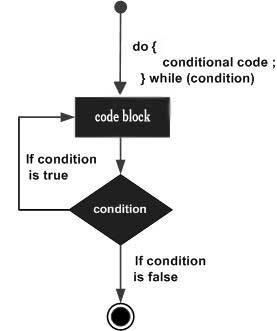Figure 8: Do while loop

Below is given the comparison chart of while and do while loop.

## Comparison Chart

while Do-while
Format while (conditional statement)

{

Statement to be executed

}

do

{

Statement to be executed

} while (conditional statement)

Position of conditional statement Conditional statement appears at the start of the loop Conditional statement appears at the end of the loop
Implementation The statement inside the loop will only be executed if the condition is true. The statement inside the loop will be executed once even if the condition is not true.
No of iterations The loop will be executed as long as the condition at the start is satisfied The loop statement will be executed as long as the condition at the end is satisfied.